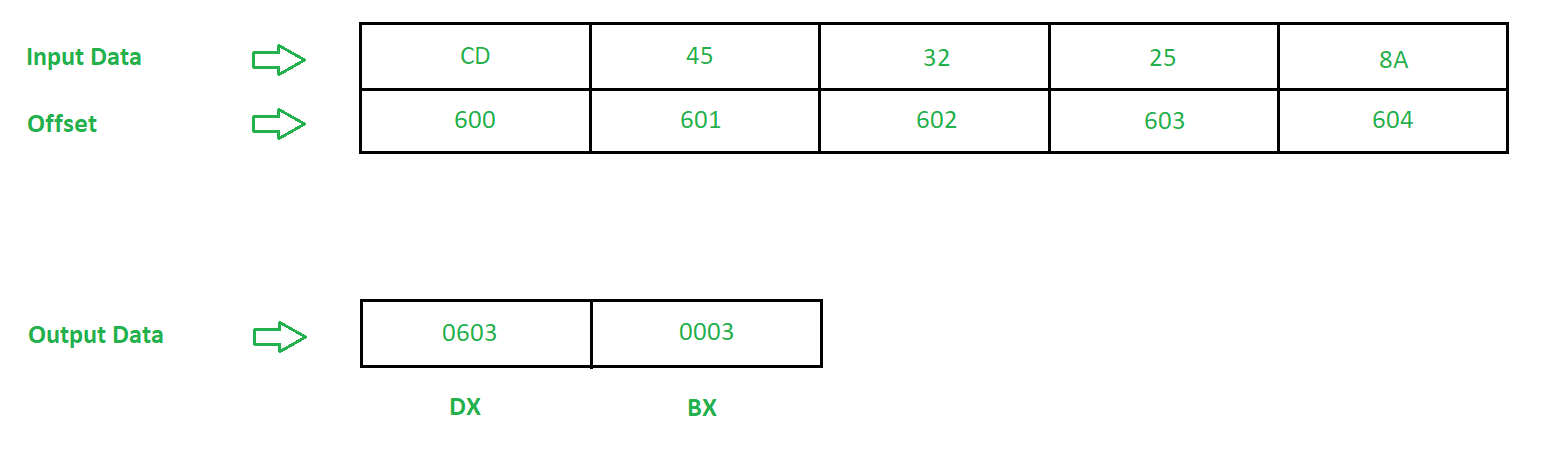GFG App
Open AppBrowser
Continue

# 8086 program to search a number in a string

Problem – Write an assembly language program in 8086 microprocessor to search a number in a string of 5 bytes, store the offset where the element is found and the number of iterations used to find the number.

Example –Algorithm –

1. Move 2000 in AX and assign it to ES
2. Assign value 600 to DI
3. Move 25 in AL
4. Move 0005 in CX
5. Move the contents of CX to BX
6. Clear the value of Directional Flag (DF)
7. Repeat step 7 till Zero Flag (ZF) is not set
8. Scan byte from [DI] and check its difference with contents of AL. Update the value of DI
9. Decrements the value of DI by 1
10. Subtract the value of BX by CX
11. Decrements the value of BX by 1
12. Halt the program

Program –

OFFSET MNEMONICS COMMENT
400 MOV AX, 2000 AX <- 2000
403 MOV ES, AX ES <- AX
405 MOV DI, 600 DI <- 600
408 MOV AL, 25 AL = 25
40A MOV CX, 0005 CX = 0005
40D MOV BX, CX BX <- CX
40F CLD DF = 0 so that memory is accessed
from lower byte to higher byte
410 REPNE SCAS B Repeat till ZF = 0. Scan value from [DI] and
compare with AL, Increment DI
414 DEC DI DI = DI – 1
415 MOV DX, DI DX = DI
417 SUB BX, CX BX = BX – CX
419 DEC BX BX = BX – 1
41B HLT Stop

Explanation –

1. MOV AX, 2000 is used to move data to register AX.
2. MOV ES, AX is used to move the data of AX to segment register ES.
3. MOV DI, 600 is used to move offset 600 to Destination Index(DI).
4. MOV AX, 25 is used to move data to AL.
5. MOV CX, 0005 is used to move data to CX.
6. MOV BX, CX is used to copy contents of CX to BX.
7. CLD is used to clear the value of Directional Flag (DF), so that memory is accessed from lower byte to higher byte.
8. REPNE SCAS B is used to scan data from DI and compare it with data from AL, if equal go to next step else increment the value of DI by 1 and repeat this step.
9. DEC DI is used to decrement contents of DI by 1.
10. MOV DX, DI is used to move the contents of DI to DX.
11. SUB BX, CX is used to subtract the contents of BX by CX.
12. DEC BX is used to decrement contents of BX by 1.
13. HLT stops executing the program and halts any further execution.
My Personal Notes arrow_drop_up